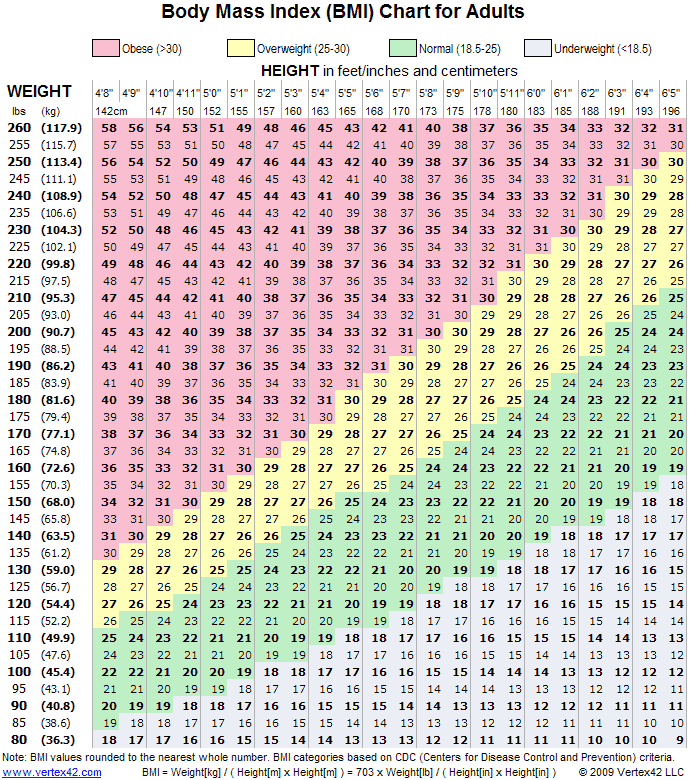Body Mass Index (BMI) Calculator - Diabetes Health Page

# Body Mass Index (BMI) Calculator

• You are here:
• Home

Body mass index (BMI) is a measure of body fat based on height and weight that applies to adult men and women.

Enter your weight and height using metric or imperial measures.``` (adsbygoogle = window.adsbygoogle || []).push({}); ```Image Source: Vertex42

To use the table, find the appropriate height in the left-hand column labeled Height. Move across to a given weight (in pounds). The number at the top of the column is the BMI at that height and weight. Pounds have been rounded off.

To use the table, find the appropriate height in the left-hand column labeled Height. Move across to a given weight (in pounds). The number at the top of the column is the BMI at that height and weight. Pounds have been rounded off.

``` (adsbygoogle = window.adsbygoogle || []).push({}); ```
``` (adsbygoogle = window.adsbygoogle || []).push({}); ```